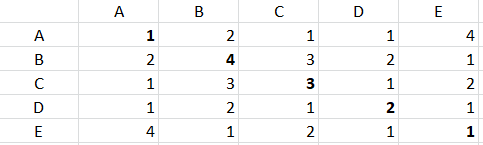# graph visualization basis symmetric matrix having values in diagonal

I have a symmetric matrix which I modified a bit:The above matrix is a symmetric matrix except the fact that I have added values in diagonal too (will tell the purpose going forward)

This matrix represents that how many times a person (A, B, C, D, E) works with other person on a publication. e.g. B and C worked 3 times together, similarly A and E worked 4 times together. Now the diagonal values represents how many times a person worked individually e.g. B worked on 4 publications (either alone or with someone else) similarly C worked on 3 publications.

Now I want to make a network analysis graph which describes relation between different person in terms of edge thickness and node size. e.g. the graph should look like this:

In graph, node circle size depends on number of publications a person worked on, e.g. circle B is largest as its diagonal value is maximum and A & E are smallest as they have lowest diagonal values. Also, the edge thickness between nodes depends on how many times they worked together, e.g. edge thickness between A & E is maximum as they worked 4 times together, compared to edge thickness (lesser than edge thickness between A & E) between B & C as they have worked 3 times together.

Is it possible to do it in Knime? Any leads would be highly appreciated

You should try looking at the Network node set, found in the ‘Other Data Types’ collection. Node size and edge thickness can each be controlled using the ‘Size Manager’ and ‘Feature Inserter’ nodes, and visualized using either a classic or JS ‘Network Viewer’ node.

There are certainly several ways to attack this problem; one might be (generally):

Create and edge table, whereby your matrix will need to be transformed such that edges are defined by each row of your table (e.g., node1, node2). A third column of the table could provide the edge weights.

A separate table can be used to define the unique nodes and their names and weights.

1 Like